#### Gail-Simon Test for Qualitative Interactions

The GAILSIMON option in the TABLES statement provides the Gail-Simon test for qualitative interaction for stratifiedtables. For details, see Gail and Simon (1985); Silvapulle (2001); Dmitrienko et al. (2005).

The Gail-Simon test is based on the risk differences in stratifiedtables, where the risk difference is defined as the row 1 risk (proportion in column 1) minus the row 2 risk. See the section Risks and Risk Differences for details. By default, the procedure uses column 1 risks to compute the Gail-Simon test. If you specify the GAILSIMON(COLUMN=2) option, the procedure uses column 2 risks.

PROC FREQ computes the Gail-Simon test statistics as described in Gail and Simon (1985),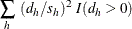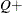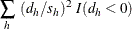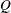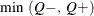where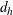is the risk difference in table h,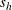is the standard error of the risk difference, and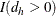equals 1 if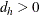and 0 otherwise. Similarly,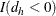equals 1 if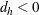and 0 otherwise. The qtables (strata) are indexed by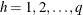.

The p-values for the Gail-Simon statistics are computed as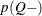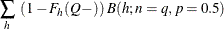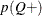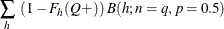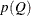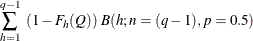where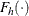is the cumulative chi-square distribution function with h degrees of freedom and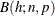is the binomial probability function with parameters n and p. The statistic Q tests the null hypothesis of no qualitative interaction. The statistictests the null hypothesis of positive risk differences. A small p-value forindicates negative differences; similarly, a small p-value forindicates positive risk differences.